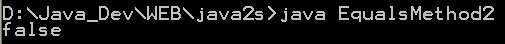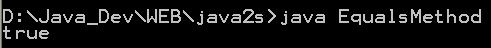# Equals « Class « Java

 1 Equals(Equal) Method2 Equals Method3 Equivalence 4 If the given objects are equal 5 Equivalence ClassSet 6 Test the equality of two object arrays 7 Compares two objects for equality, where either one or both objects may be null 8 This program demonstrates the equals method